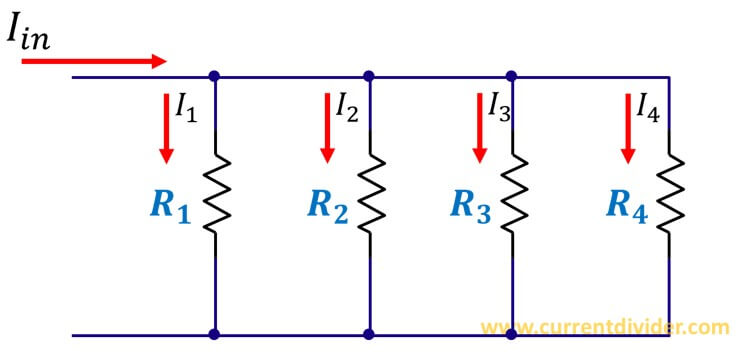# Current Divider

Learn everything about Current Division in Parallel Circuits.

The current divider rule (CDR) is one of most basic rules of Electrical Engineering. Today you'll learn to apply the CDR to four parallel resistors.

Let's consider a circuit with 4 parallel resistors.The application of CDR in this circuit requires two steps:
1. Find the equivalent resistance of four resistors
2. Apply the basic CDR formula.
Solving four parallel resistors is very easy and is similar to the two parallel resistors.